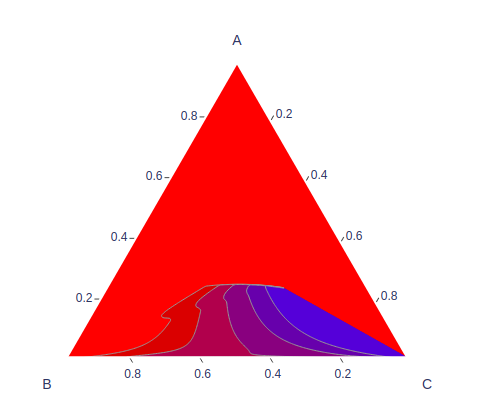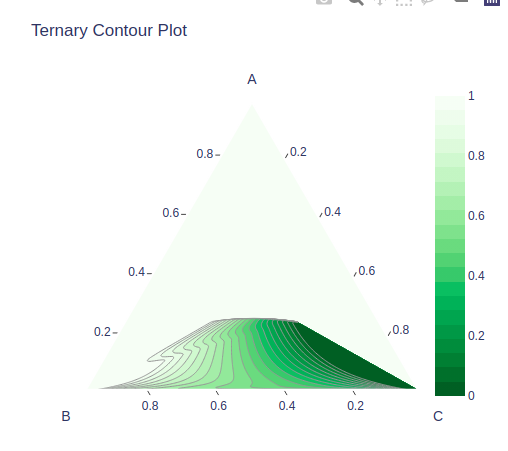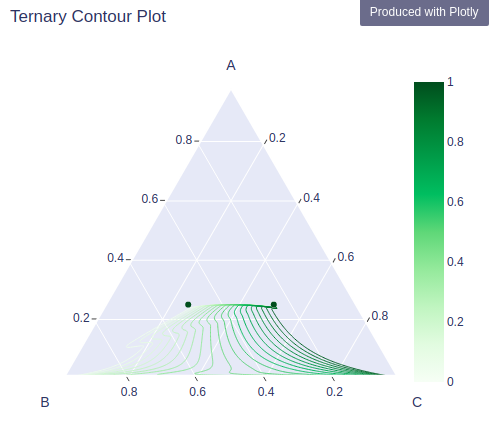# Ternary contours Plot using Plotly in Python

A Plotly is a Python library that is used to design graphs, especially interactive graphs. It can plot various graphs and charts like histogram, barplot, boxplot, spreadplot, and many more. It is mainly used in data analysis as well as financial analysis. plotly is an interactive visualization library.

## Ternary contours in Plotly

In plotly, ternary contours plot can be made by using the create_ternary_contour method of figure_factory class which helps to represent the isovalues lines which are defined inside the ternary diagram, where the sum of three variables is constant. It graphically depicts the ratios of the three variables as positions in an equilateral triangle.

Syntax: create_ternary_contour(coordinates, values, pole_labels=[‘a’, ‘b’, ‘c’],ncontours=None,  interp_mode=’ilr’, showmarkers=False)

Example:

## Python3

 `import` `plotly.figure_factory as ff ` `import` `numpy as np ` ` `  `test_data ``=` `np.array([[``0``, ``0``, ``1``, ``0``], ` `                      ``[``0.25``, ``0.25``, ``0.5``, ``0``], ` `                      ``[``0.25``, ``0.25``, ``0.5``, ``0``], ` `                      ``[``0.25``, ``0.25``, ``0.5``, ``1``], ` `                      ``[``0.25``, ``0.5``, ``0.25``, ``1``], ` `                      ``[``0``, ``1``, ``0``, ``1``]]) ` ` `  `# barycentric coords: (a,b,c) ` `a ``=` `test_data[:, ``0``] ` `b ``=` `test_data[:, ``1``] ` `c ``=` `test_data[:, ``2``] ` ` `  `# values is stored in the last column ` `v ``=` `test_data[:, ``-``1``] ` ` `  `fig ``=` `ff.create_ternary_contour( ` `    ``np.array([a, b, c]), v, ` `    ``pole_labels``=``[``'A'``, ``'B'``, ``'C'``], ` `) ` ` `  `fig.show() `

Output:## Customizing ternary contour plot

Height, width, colorscale, and many other values can be customized by using the parameter provided by this function.

Example:

## Python3

 `import` `plotly.figure_factory as ff ` `import` `numpy as np ` ` `  `test_data ``=` `np.array([[``0``, ``0``, ``1``, ``0``], ` `                      ``[``0.25``, ``0.25``, ``0.5``, ``0``], ` `                      ``[``0.25``, ``0.25``, ``0.5``, ``0``], ` `                      ``[``0.25``, ``0.25``, ``0.5``, ``1``], ` `                      ``[``0.25``, ``0.5``, ``0.25``, ``1``], ` `                      ``[``0``, ``1``, ``0``, ``1``]]) ` ` `  `# barycentric coords: (a,b,c) ` `a ``=` `test_data[:, ``0``] ` `b ``=` `test_data[:, ``1``] ` `c ``=` `test_data[:, ``2``] ` ` `  `# values is stored in the last column ` `v ``=` `test_data[:, ``-``1``] ` ` `  `fig ``=` `ff.create_ternary_contour( ` `    ``np.array([a, b, c]), v, ` `    ``pole_labels``=``[``'A'``, ``'B'``, ``'C'``], ` `    ``ncontours``=``20``, ` `    ``colorscale``=``'Greens'``, ` `    ``showscale``=``True``, ` `    ``title``=``'Ternary Contour Plot'` `) ` ` `  `fig.show() `

Output:## Ternary contour plot with data points and lines

Only lines can be shown by the parameter coloring and setting it to lines and data points can be shown by passing True to the showmarkers parameter.

Example:

## Python3

 `import` `plotly.figure_factory as ff ` `import` `numpy as np ` ` `  `test_data ``=` `np.array([[``0``, ``0``, ``1``, ``0``], ` `                      ``[``0.25``, ``0.25``, ``0.5``, ``0``], ` `                      ``[``0.25``, ``0.25``, ``0.5``, ``0``], ` `                      ``[``0.25``, ``0.25``, ``0.5``, ``1``], ` `                      ``[``0.25``, ``0.5``, ``0.25``, ``1``], ` `                      ``[``0``, ``1``, ``0``, ``1``]]) ` ` `  `# barycentric coords: (a,b,c) ` `a ``=` `test_data[:, ``0``] ` `b ``=` `test_data[:, ``1``] ` `c ``=` `test_data[:, ``2``] ` ` `  `# values is stored in the last column ` `v ``=` `test_data[:, ``-``1``] ` ` `  `fig ``=` `ff.create_ternary_contour( ` `    ``np.array([a, b, c]), v, ` `    ``pole_labels``=``[``'A'``, ``'B'``, ``'C'``], ` `    ``ncontours``=``20``, ` `    ``colorscale``=``'Greens'``, ` `    ``showscale``=``True``, ` `    ``title``=``'Ternary Contour Plot'``, ` `    ``coloring``=``'lines'``, ` `    ``showmarkers``=``True` `) ` ` `  `fig.show() `

Output:Attention geek! Strengthen your foundations with the Python Programming Foundation Course and learn the basics.

To begin with, your interview preparations Enhance your Data Structures concepts with the Python DS Course.

My Personal Notes arrow_drop_upIf you like GeeksforGeeks and would like to contribute, you can also write an article using contribute.geeksforgeeks.org or mail your article to contribute@geeksforgeeks.org. See your article appearing on the GeeksforGeeks main page and help other Geeks.

Please Improve this article if you find anything incorrect by clicking on the "Improve Article" button below.

Article Tags :

Be the First to upvote.

Please write to us at contribute@geeksforgeeks.org to report any issue with the above content.